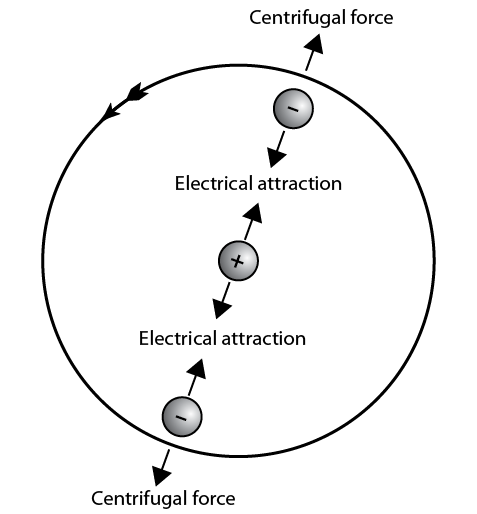# 1.1: Basic principles

•• Camosun College
$$\newcommand{\vecs}{\overset { \rightharpoonup} {\mathbf{#1}} }$$ $$\newcommand{\vecd}{\overset{-\!-\!\rightharpoonup}{\vphantom{a}\smash {#1}}}$$$$\newcommand{\id}{\mathrm{id}}$$ $$\newcommand{\Span}{\mathrm{span}}$$ $$\newcommand{\kernel}{\mathrm{null}\,}$$ $$\newcommand{\range}{\mathrm{range}\,}$$ $$\newcommand{\RealPart}{\mathrm{Re}}$$ $$\newcommand{\ImaginaryPart}{\mathrm{Im}}$$ $$\newcommand{\Argument}{\mathrm{Arg}}$$ $$\newcommand{\norm}{\| #1 \|}$$ $$\newcommand{\inner}{\langle #1, #2 \rangle}$$ $$\newcommand{\Span}{\mathrm{span}}$$ $$\newcommand{\id}{\mathrm{id}}$$ $$\newcommand{\Span}{\mathrm{span}}$$ $$\newcommand{\kernel}{\mathrm{null}\,}$$ $$\newcommand{\range}{\mathrm{range}\,}$$ $$\newcommand{\RealPart}{\mathrm{Re}}$$ $$\newcommand{\ImaginaryPart}{\mathrm{Im}}$$ $$\newcommand{\Argument}{\mathrm{Arg}}$$ $$\newcommand{\norm}{\| #1 \|}$$ $$\newcommand{\inner}{\langle #1, #2 \rangle}$$ $$\newcommand{\Span}{\mathrm{span}}$$$$\newcommand{\AA}{\unicode[.8,0]{x212B}}$$

Explain fundamentals of electricity

Over the centuries scientists have discovered that electricity is predictable and measurable. Being familiar with the fundamentals of electricity will help you to understand how and why electrical circuits work.

Basic principles

Electricity is a form of energy. To understand electricity, it is important that you first understand the structure of matter. Anything that occupies space and has weight is called matter. All liquids, gases, and solids are examples of matter in different forms. Matter is made of smaller units called atoms. Atoms can be grouped together in compounds to form molecules.

Atomic theory

Atoms are the most basic part of matter and differ in atomic structure from each other. The structure of the atom can be described in much the same way as the solar system. Instead of the Sun at the centre, there is a nucleus. This nucleus is made of two basic particles: protons and neutrons.

Neutrons make up the mass (or weight equivalency) of the atom, have no electrical charge, and are considered to be neutral. Protons are particles that have a positive (+) electrical charge and cannot be separated from the nucleus. Surrounding the nucleus in orbits are electrons. These are tiny particles with a negative (–) electrical charge. Figure 1 shows a model of a carbon atom.Figure $$\PageIndex{1}$$: (CC BY-NC-SA; BC Industry Training Authority)
1. Carbon atomFigure $$\PageIndex{1}$$: (CC BY-NC-SA; BC Industry Training Authority)
2. Hydrogen and copper atomsFigure $$\PageIndex{1}$$: (CC BY-NC-SA; BC Industry Training Authority)
3. Electrical attractionFigure $$\PageIndex{1}$$: (CC BY-NC-SA; BC Industry Training Authority)
4. Transmission of impulse

Sources of electrical force

You have just learned that if there is a surplus of electrons at one end of a conductor and a deficiency at the other end, a current flows in the conductor. There are devices that create this difference in charge so that a current will flow. These devices are referred to as sources of electromotive force. These sources include:

• chemical
• electromagnetic induction
• friction
• heat
• pressure
• light

Chemical

A battery is a source of electrical force due to the chemical reaction that takes place between plates and an electrolyte. This reaction causes a buildup of positive ions on one plate and negative ions on the other plate. This electrical difference between the plates is also known as potential difference.

Electromagnetic induction

Electric force can be generated by using a magnetic field. This is the method by which most of the electrical energy we use is produced. An example is an alternator or generator.

Friction

Friction can cause free electrons to move from one body to another and be stored there temporarily. When you walk across a carpet, electrons are transferred to the atoms in your body and you return them to other atoms when you touch a metallic object.

Heat

If two unlike metals are placed together and heated, they will produce electrical force. An example is the thermocouple in a furnace.

Pressure

Certain crystals will produce electricity if they are squeezed under extreme pressure. An example is a barbecue starter (also called piezoelectric generator).

Light

Some crystals and semiconductors will produce electrical force when they are exposed to light. An example is the photocell in a calculator.

All six of these sources of EMF achieve the same thing. They separate charge by:

• imparting energy to the electrons
• pushing them against an electrostatic field
• causing a surplus of electrons (negative charge) at one terminal of the source and a deficiency of electrons (positive charge) at the other terminal

In a sense, the process can be likened to compressing a spring. The energy stored in the compressed spring can be used later to do useful work. The same is true of the separated charges: they store energy that can be used to do useful work.

Electrical energy always comes from some other form of energy. The source of EMF is simply the device that makes the conversion from some other form of energy to electrical energy.